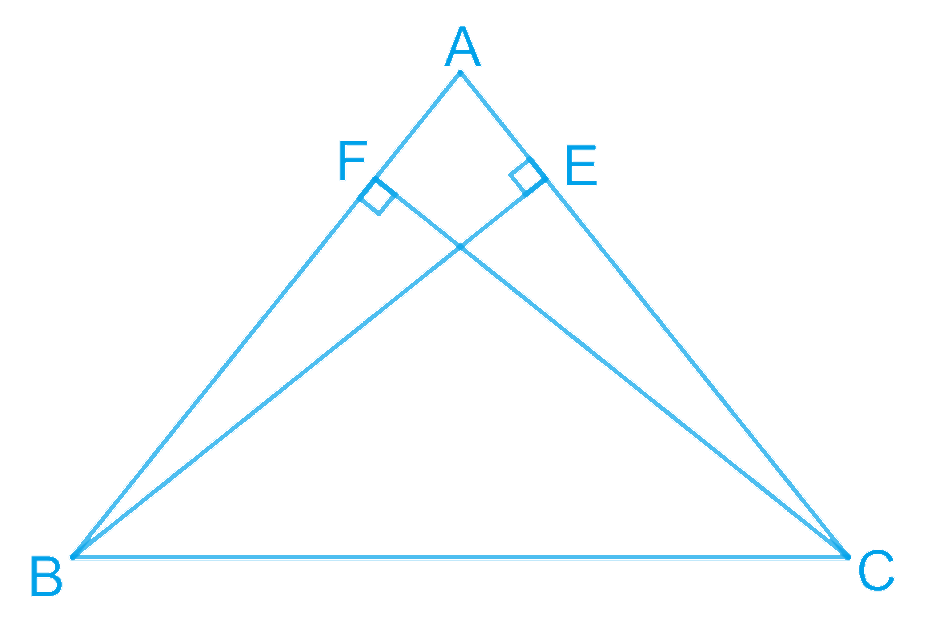# Ex.7.2 Q3 Triangles Solution - NCERT Maths Class 9

Go back to  'Ex.7.2'

## Question

$$ABC$$ is an isosceles triangle in which altitudes $$BE$$ and $$CF$$ are drawn to equal sides $$AC$$ and $$AB$$ respectively (see the given figure). Show that these altitudes are equal.

Video Solution
Triangles
Ex 7.2 | Question 3

## Text Solution

What is known?

Sides $$AB=AC,$$

$$BE\bot AC\ \text{and CF}\bot \text{AB}$$

To prove:

Altitudes $$BE$$ and $$CF$$ are equal or $$BE = CF$$

Reasoning:

We can show two triangles $$ABE$$ and $$ACF$$ congruent by using AAS congruency rule and then we can say corresponding parts of congruent triangles will be equal.Steps:

$$\text{In } \Delta AEB \text{ and } \Delta AFC,$$

\begin{align}& \angle AEB = \angle AFC \;(\text{Each }90^{\circ}) \\ & \angle A = \angle A\; (\text{Common angle}) \\ & AB = AC\; (\text{Given}) \\ & \therefore \Delta AEB \cong \Delta AFC \\& \text{(By AAS congruence rule)} \end{align}

$$\therefore BE = CF$$ (By $$CPCT$$ )

Video Solution
Triangles
Ex 7.2 | Question 3

Learn from the best math teachers and top your exams

• Live one on one classroom and doubt clearing
• Practice worksheets in and after class for conceptual clarity
• Personalized curriculum to keep up with school